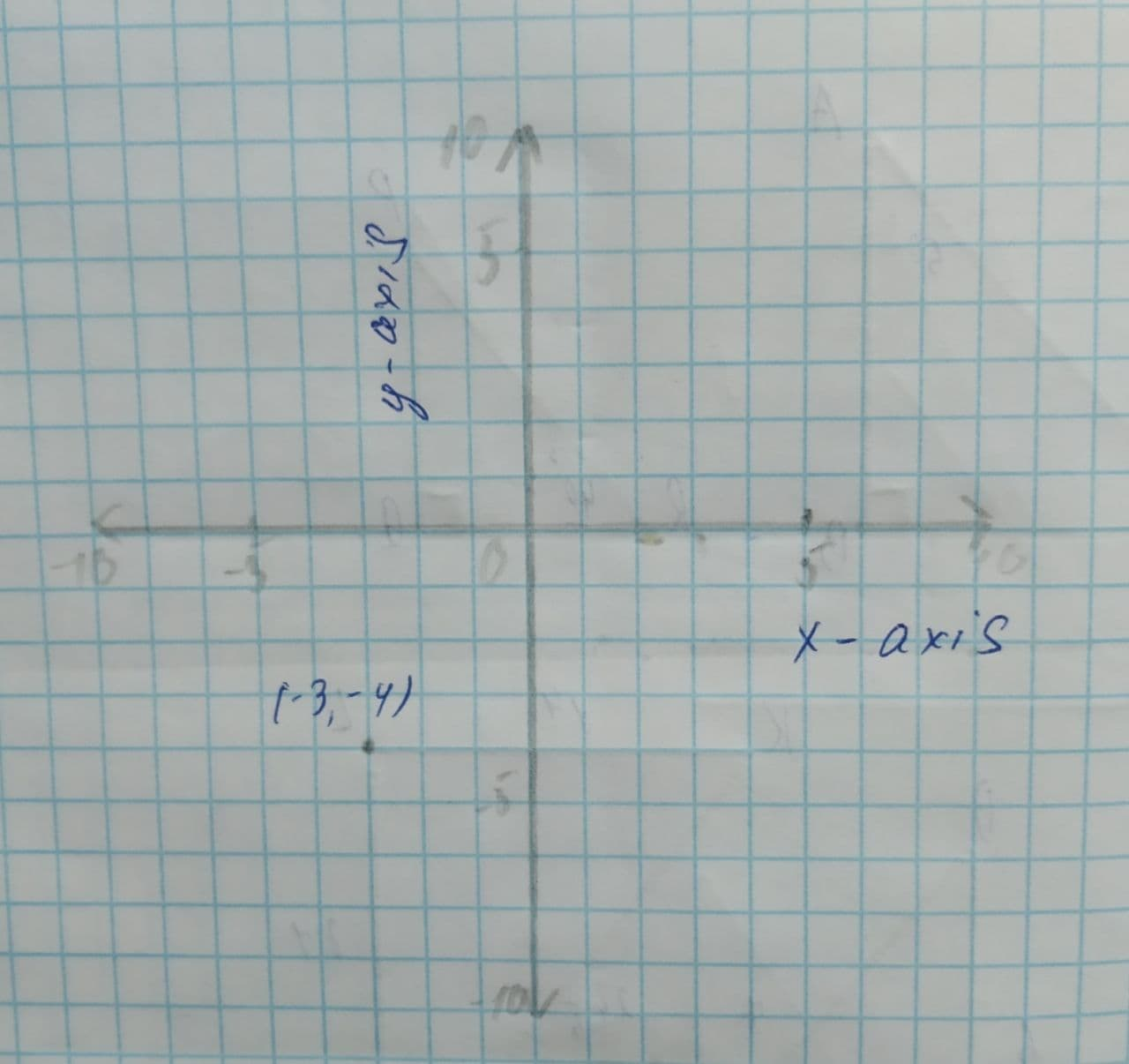Question# Plot the complex number z = -3 - 4i and find its absolute value.

Piecewise-Defined Functions
ANSWEREDPlot the complex number $$z = -3 - 4i$$ and find its absolute value.2021-09-09

Step 1:To determine
Plot the complex number $$z=−3−4i$$ and find its absolute value.
Step 2:Calculation
The given complex number $$z=−3−4i$$ can be plotted as the cooordinate $$(−3,−4)$$ on the x-y plane.
So, the complex number is plotted as:Consider the complex number $$z=x+iy$$.
The absolute value is given by:
$$\displaystyle{\left|{z}\right|}=\sqrt{{{x}^{{{2}}}+{y}^{{{2}}}}}$$
Consider the given complex number $$z=−3−4i$$
So, the absolute value is given by:
$$\displaystyle{\left|{z}\right|}=\sqrt{{{\left(-{3}\right)}^{{{2}}}+{\left(-{4}\right)}^{{{2}}}}}$$
$$\displaystyle\Rightarrow{\left|{z}\right|}=\sqrt{{{9}+{16}}}$$
$$\displaystyle\Rightarrow{\left|{z}\right|}=\sqrt{{{25}}}$$
$$\displaystyle\Rightarrow{\left|{z}\right|}={5}$$